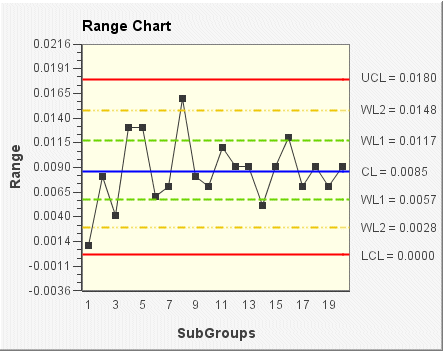# Range chart

The Range chart is a control chart that shows how the range (that is, the difference between the smallest and largest values) of subgroups changes over time. This chart is typically used with smaller subgroup sizes (less than 25). For larger subgroup sizes, or for variable subgroups, the Sigma chart is recommended.

In the following Range chart example, the subgroup size for the sample data is 5 (the default size). The range of each subgroup is plotted on the chart. The control limits (shown on the chart as UCL, CL, LCL, WL1, and WL2) are set according to the data and a sigma limit of 3.